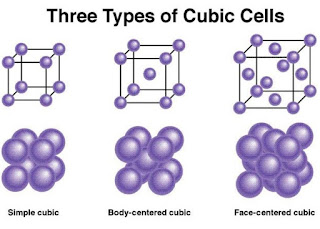# IMPORTANT QUESTIONS OF SOLID STATE CHEMISTRY FOR WBCHSE CLASS 12

May 7, 2020

Last Updated on January 23, 2023 by Science Master

## Important Questions of Solid State Chemistry

### Class-12

The solid state chapter is the first and most important chapter in the twelfth grade chemistry. Here is a discussion of the classification of solids and the various defects of Crystal.

Below are some important questions from Solid State that will help students prepare for exams. From here M.C.Q and S. A. Q. Both types of questions are important.## Important Questions of Solid State Chemistry

1)   What is crystal?

2) What is   crystal lattice or lattice lattice?

3)   What is the lattice point?

4)   What is a single cell? Write the name of different types of
the three-dimensional unit cell.

5)
Why  Glass is called super cooled viscous liquid ?

6)   Arrange different types of cubic single cells  according to the number of particles.

7) The   atomic mass of an element is M. How many single cells will there be in the amount of x grams of the element  , if the single  cell is face centeerd cube.

8)   What is braves lattice?

9)   What is meant by single cell packing efficiency.

10) Determine the packing efficiency of simple unit cell and the void space of unit cell.

11) Determine the packing efficiency of face centered unit cell and void space of unit cell.

12) Determine the packing efficiency of the body-centered unit cell and the space of the unit cell.

13)  Simple, body-centered  and face centered cube cell forming a single particle radius, respectively, r1 , r 2 , r3 .  The length of the edge of a single cell is “a”. Then what is the ratio of  r1 , r2 , r3.

14) If the number of atoms in a single cell of an element crystal is ‘z’ edge length  ‘a’ and the molecular mass of the element is M, determine the density of the crystal (þ).

15)  A metal  forms
face – centered cubic   crystal. If the atomic radius of the metal is 1.28, what is the length of the edge of a unit cell?

16)  Led crystals are face centered cube in nature and have a density of 11.35 gcm -3 . Determine the radius of the atom of lead. (M = 207)

17) Cr (52) forms body-centered cubic crystals. If the edge length of a unit cell is 287pm, determine the radius and density of the Cr atom.

18) The   length of the edge of a body-centered unit cell of an element is 288 pm, the density of the metal is 7.2 gcm -3 .  How many atoms and how many unit cells are there in the amount of 208 grams of that element .

19) The concentration of an
element is  2.8 gcm -3 . It is  face centered cubic cell and the length of the edge of the 400  pm. Determine the atomic mass of the element.

20)  What is a point defect?

21) What is a Scottish defect?

22) What is a Frankel defect?

23) Write the difference between Scotch defect and Frankel defect.

24) What is doping?

25) Why NaCl turns yellow when NaCl is heated in the presence of sodium vapor?

26) Why does ZnO turn yellow when heated?

27) Why is the conductivity increased when ZnO is heated?

28) What is the Co-ordination  number?

29) What is the total number of fcc crystals?

30) Find the ratio of the radius of the octagonal gap.

31) What is the amount of cation void in the crystal as  a result of doping 10 -3 mol% SrCl 2 in NaCl crystal ?

32) The  structure of a binary solid (A + B  ) is similar to that

of a zinc blend.  B ions form lattice and A ions fill 25% of the
quadratic gap. Determine the signal of the solid.

33) The
metal oxide x O y is formed  cubic crystal by  O2 atomsIn that,  2/3  of the octahedral gaps are occupied by M atoms. But find the values ​​of x and y.

34) What is the  F-centre?

35) What is waste semiconductor?

36) What is n type semiconductor?

37) What is  type semiconductor?

MCQ and SAQ Type question  of solid state

1)   What is mixed with pure Si in  type semiconductor?

2) In   which crystal class body-centered lattice is not possible?

3) What kind of substance shows anti ferromagnetic properties?

4)  a ≠ b  ≠ c and 𝛼 ≠ 𝛽 ≠ 𝛾 ≠ 90 Which crystal?

5) In which case the cations are located internally?

6) What is the amount of space in fcc and bcc crystals?

7) What is the number of particles in fcc and bcc unit cell?

8) What type of semiconductor is formed by mixing a small amount of As with pure Si?

9) What is the number of co-ordination of face centered unit cell?

10) What kind of defects does not cause any change in the density of the crystal?

11) Write the name of an addition that shows both Scottish defect and Frankel defect.

12) Arrange simple, body-centered, face-centered cubic  unit cells according to packing skills.

13) Which of ferromagnetic and paramagnetic can be turned into a permanent magnet.

14) What kind of defects increases the density of the crystal?

15)  Which of the following Scottish defect and Frankel defect decreases the density of crystal?

16) The highest packing efficiency can be seen in any of the following – bcc / fcc / scc.

17) The lattice point of pure crystal cannot contain any of the following – atoms /  molecules / ions / electrons.